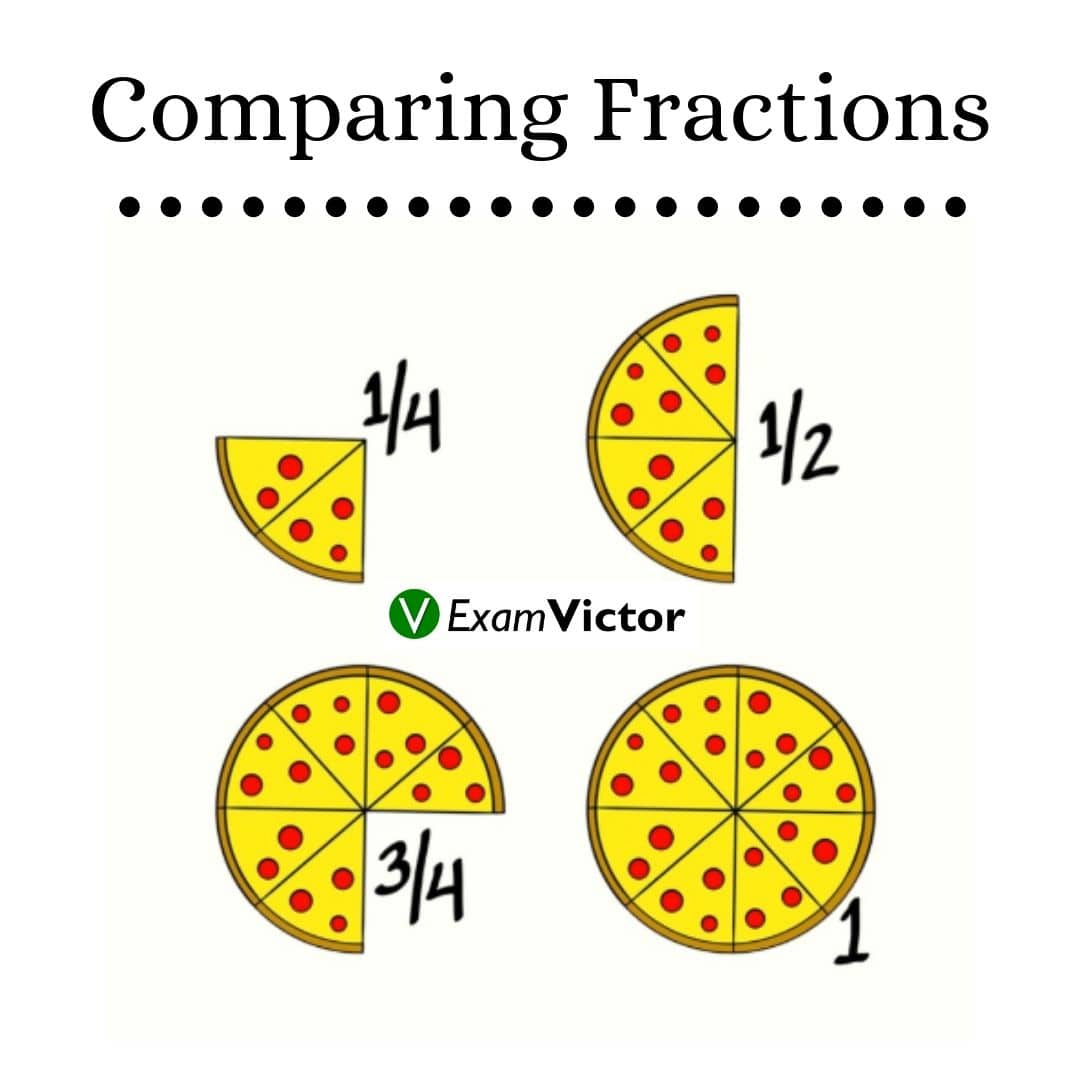# Comparing FractionsLast Updated: Nov 5, 2020

• Fraction = Number of Parts/ Total Parts
• Every fraction has a numerator that equals the number of parts we have and a denominator equaling the total number of parts in a whole
• Any Number Can Be Written As a Fraction
• Write any whole number over 1 to make it a fraction since the total number of parts in any undivided whole is one
• Multiplying fractions is easy, just multiply straight across

Rules for Comparing Fractions

1. Relation: Like Denominators

How To Compare: Look at the numerators. The larger fraction is the one with the greater numerator.

Example: 3/5 > 1/5

2. Relation: Unlike Denominators

How To Compare: Convert each fraction to an equivalent fraction with a common denominator. The larger fraction is the one with the greater numerator.

​Example: 5/8 < 7/10  since 25/40 < 28/40

3. Relationship: Like Numerators

How To Compare: Look at the denominators. The fraction with the smaller denominator is the larger fraction.

​Example: 2/7  > 2/9

Equivalent Fractions: fractions that represent the same quantity.

Fundamental Law of Fractions: the value of a fraction does not change when its numerator and denominator are both multiplied by the same number (not zero).

Simplifying Fractions: reducing fractions. To simplify (or reduce) a fraction, find a number that will divide exactly into BOTH the numerator and the denominator. Try to find the "largest" such value (the greatest common divisor).

Always remember that:
1)  If both numbers end in 0 and/or 5, they are divisible by 5.
2)  If both numbers are such that the sum of the digits of the number
is divisible by 3, then the numbers are divisible by 3.
3)  If both numbers end in zeros, they are divisible by 10.
4)  If both numbers are even, they are divisible by 2.
5)  If both numbers end in 25, 50, 75 or 100, they are divisible by 25.

This video lays out the basics of comparing fractions. Understanding this topic well is necessary to solve questions that are asked in various competitive exams in the Quant and DI sections - applicable to CAT, XAT, MAT, SNAP, IIFT, CLAT, AILET, DU LLB, any other entrance exam as well.